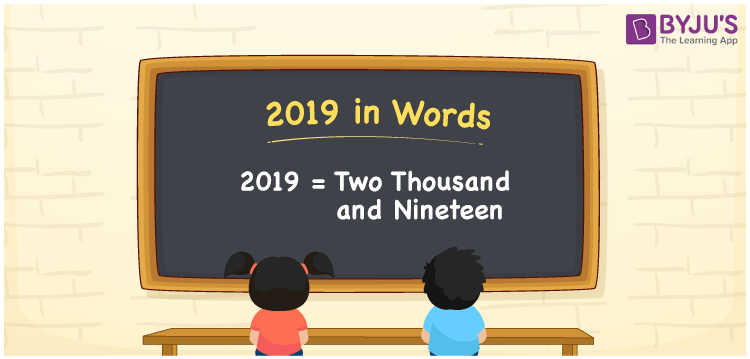# 2019 in words

2019 in words is written as Two Thousand and Nineteen. 2019 represents the count or value. The article on Counting Numbers can give you an idea about count or counting. The number 2019 is used in expressions that relate to money, distance, length, year and others. Let us consider an example for 2019. “The first case of Covid was detected in the year Two Thousand and Nineteen.” Another example, “The total number of students from all the sections of grade 1 are Two Thousand and Nineteen.”

 2019 in words Two Thousand and Nineteen Two Thousand and Nineteen in Numbers 2019

## 2019 in English Words## How to Write 2019 in Words?

We can convert 2019 to words using a place value chart. This can be done as follows. The number 2019 has 4 digits, so let’s make a chart that shows the place value up to 4 digits.

 Thousands Hundreds Tens Ones 2 0 1 9

Thus, we can write the expanded form as:

2 × Thousand + 0 × Hundred + 1 × Ten + 9 × One

= 2 × 1000 + 0 × 100 + 1 × 10 + 9 × 1

= 2019

= Two Thousand and Nineteen.

2019 is the natural number that is succeeded by 2018 and preceded by 2020.

2019 in words – Two Thousand and Nineteen.

Is 2019 an odd number? – Yes.

Is 2019 an even number? – No.

Is 2019 a perfect square number? – No.

Is 2019 a perfect cube number? – No.

Is 2019 a prime number? – No.

Is 2019 a composite number? – Yes

## Solved Example

1. Write the number 2019 in expanded form

Solution: 2 × 1000 + 0 × 100 + 1 × 10 + 9 × 1

We can write 2019 = 2000 + 000 + 10 + 9

= 2 × 1000 + 0 × 100 + 1 × 10 + 9 × 1

## Frequently Asked Questions on 2019 in words

Q1

### How to write 2019 in words?

2019 in words is written as Two Thousand and Nineteen.
Q2

### State whether True or False. 2019 is divisible by 2?

False. 2019 is not divisible by 2.
Q3

### Is 2019 divisible by 10?

No. 2019 is not divisible by 10.